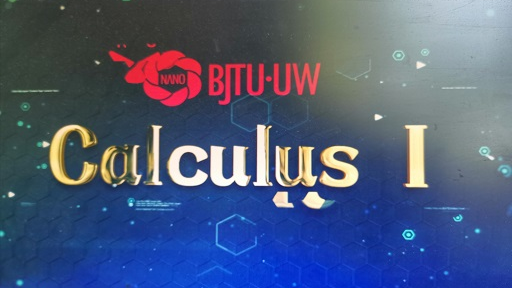# Calculus Ⅰ

Practice more, learn more! Wish you enjoy this course and benifit from our lectures.

## Calculus Ⅰ课程简介：前往报名学习## Calculus Ⅰ课程简介：

Practice more, learn more! Wish you enjoy this course and benifit from our lectures.

## Calculus Ⅰ课程目录：

#### Functions and Models

--Four Ways to Represent a Function

--Mathematical Models:A Catalog of Essential Functions. New Functions from Old Functions

--Exponential Functions, Inverse Functions and Logarithms

#### Limits and Derivatives

--The Limit of a Function. Calculating Limits Using the Limits Laws

--The Precise Definition of a Limit. Continuity

--Limits at Infinity;Horizontal Asymptotes

--Derivatives and Rates of Change. The Derivative as a Function

#### Differentiation Rules

--Derivatives of Polynomials and Exponential Functions

--The Product and Quotient Rules

--Derivatives of Trigonometric Functions

--The Chain Rule，Implicit Differentiation

--Derivatives of Logarithmic Functions

--Exponential Growth and Decay

--Related Rates

--Linear Approximations and Differentials

--Hyperbolic Functions

#### Applications of Differentiation

--Maximum and Minimum Values

--The Mean Value Theorem

--How Derivatives Affect the Shape of a Graph

--Indeterminate Froms and 1'Hospital's Rule

--Optimization Problems

--Newton's Method

--Antidervatives

#### Integrals

--Areas and Distances，The Definite Integral

--The Fundamental Theorem of Calculus

--Indefinite Integrals and the Net Change Theorem

--The Substitution Rule

#### Applications of Integration

--Areas Between Curves

--Volumes

--Volumes by Cylindrical Shells

--Work

--Average Value of a Function

#### Techniques of Integration

--Integration by Patrs

--Trigonometric Integral

--Trigonometric Substitution

--Integration of Rational Functions by Parital Fractions

--Approximate Integration

--Improper Integrals

#### Further Applications of Integration

--Arc Length

--Area of a Surface of Revolution

--Applications to Physics and Engineering

#### Differential Equations

--Modeling with Differential Equations

--Separable Equations

--Linear Equations

#### Parametric Equations and Polar Coordinates

--Curves Defined by Parametric Equations

--Calculus with Parametric Curves

--Polar Coordinates

--Areas and Lengths in Polar Coordinates

## Calculus Ⅰ授课教师：

### 吴发恩-教授-北京交通大学-理学院1994获得中科院数学所博士学位，同年到北京交大工作。现任交大理学院数学系教授。研究方向为微分几何。曾主持一项国家自然科学基金项目。在中国科学，数学学报，美国数学会会报等国内外重要刊物上发表论文十多篇。曾到加拿大麦克马斯特大学，美国纽约理工大学做访问学者。多年来，除了承担交大国际班的微积分全英文教学，还结合自己的专业方向，承担数学系数学与运用数学的专业课《微分几何》与《点集拓扑》的教学任务。

© 柠檬大学 2020